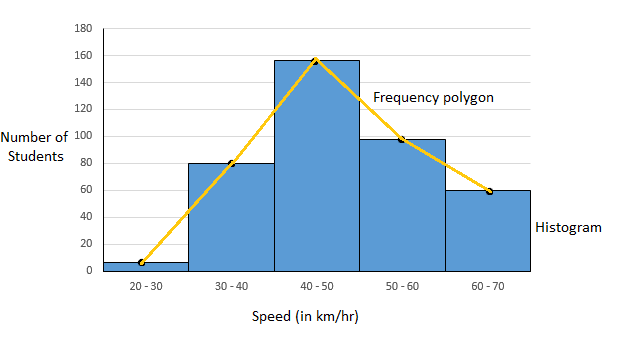# Maharashtra (MSBSHSE) SSC Board Question Paper For Class 10 Maths Paper 2 (Algebra) 2015 In PDF

## Maharashtra SSC Maths Algebra (Paper 2) Exam Question Paper 2015 with Solutions – Free Download

MSBSHSE (Maharashtra board) SSC Class 10 maths 2015 Algebra Question Paper with solutions are available at BYJU’S, which can be accessed and downloaded in a pdf format. Algebra plays an important role in everyday life, when dealing with different situations. For example, calculating interest, buying and selling goods for a certain price and so on. In all these situations we generally use algebraic calculations, knowing or unknowingly. Hence, students should focus more on these concepts. Maharashtra board Class 10 Maths Algebra chapters cover all such topics, which are necessary for higher education as well as real-life situations. Students can also download MSBSHSE SSC Class 10 Maths previous year question papers to practise for the board exam.

### MSBSHSE Class 10 Mathematics Question Paper 2015 Algebra Paper with Solutions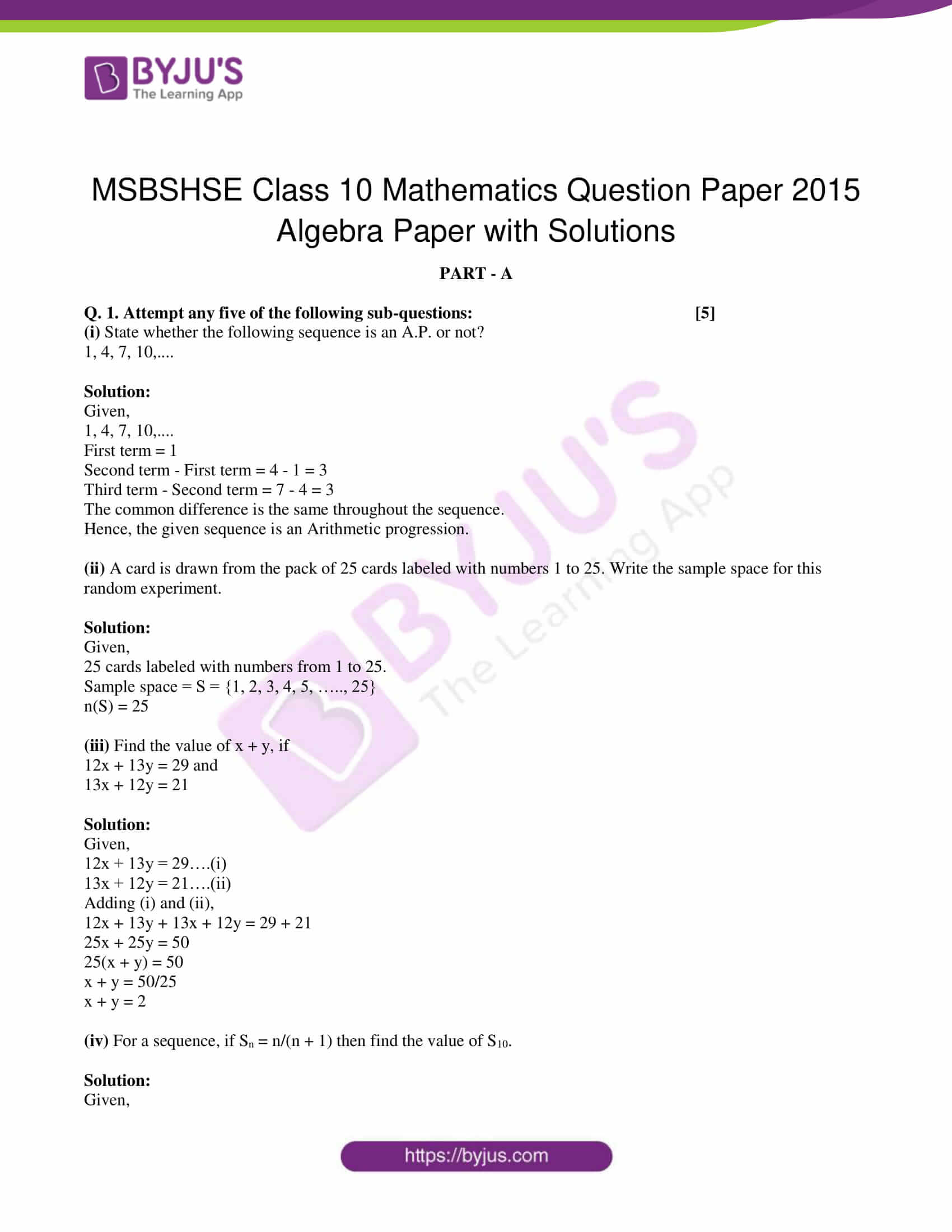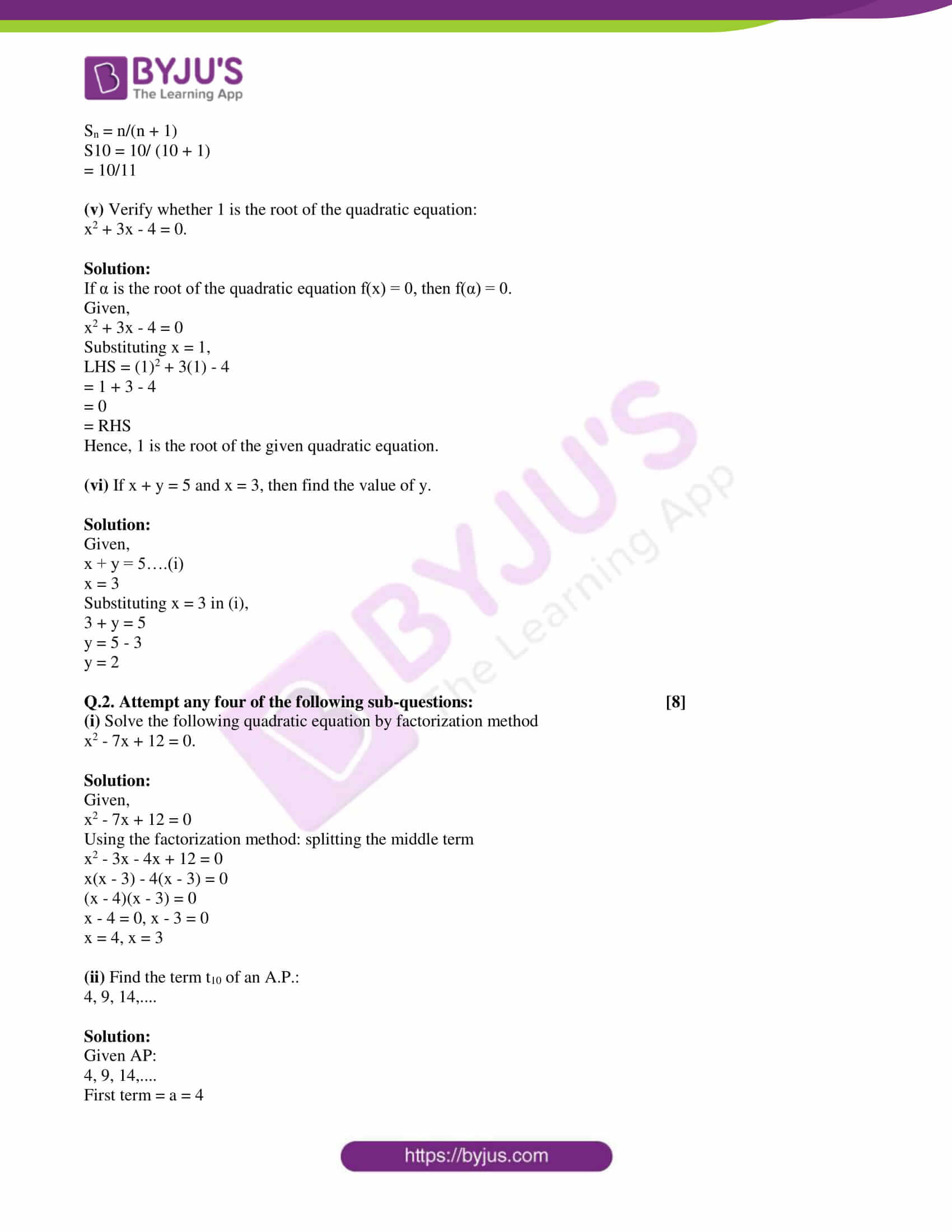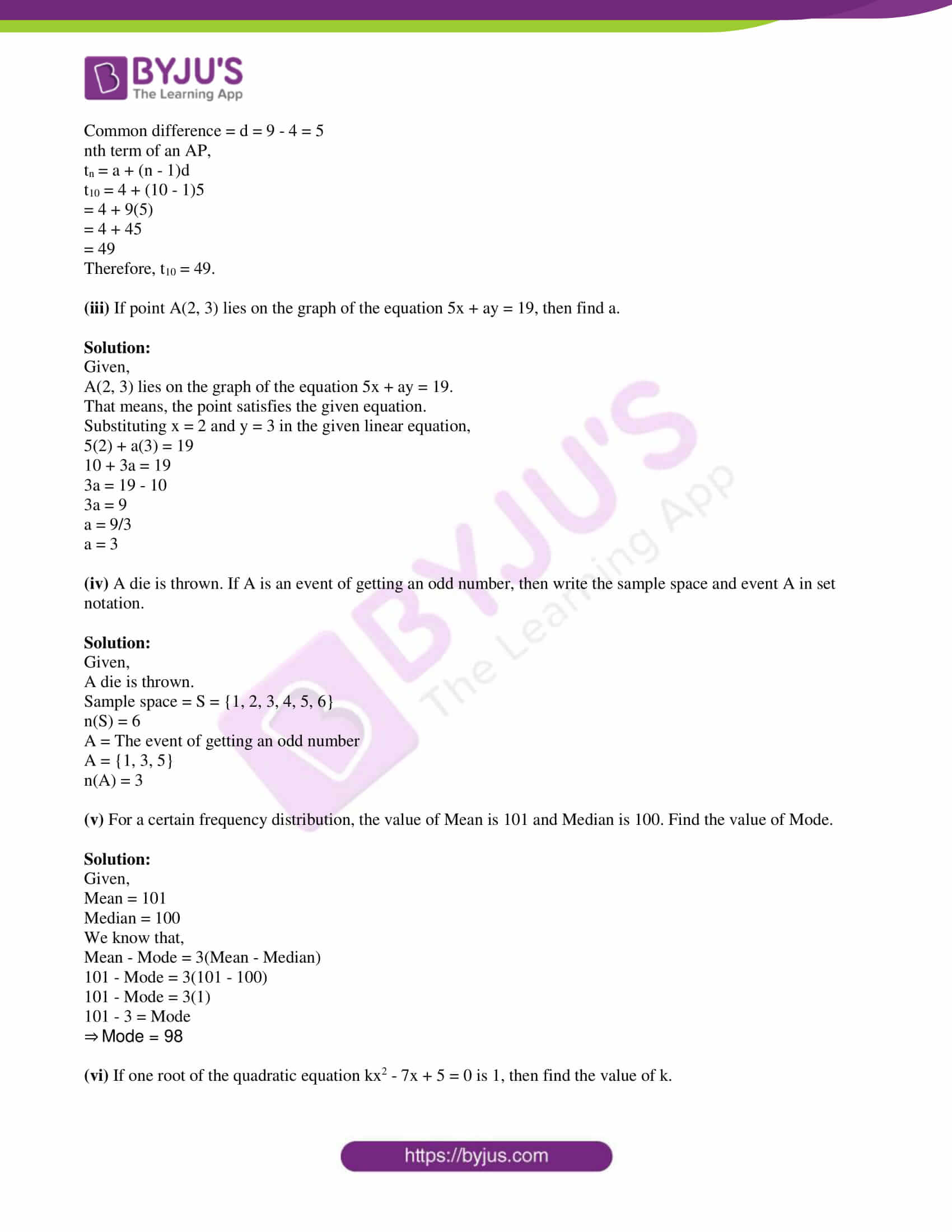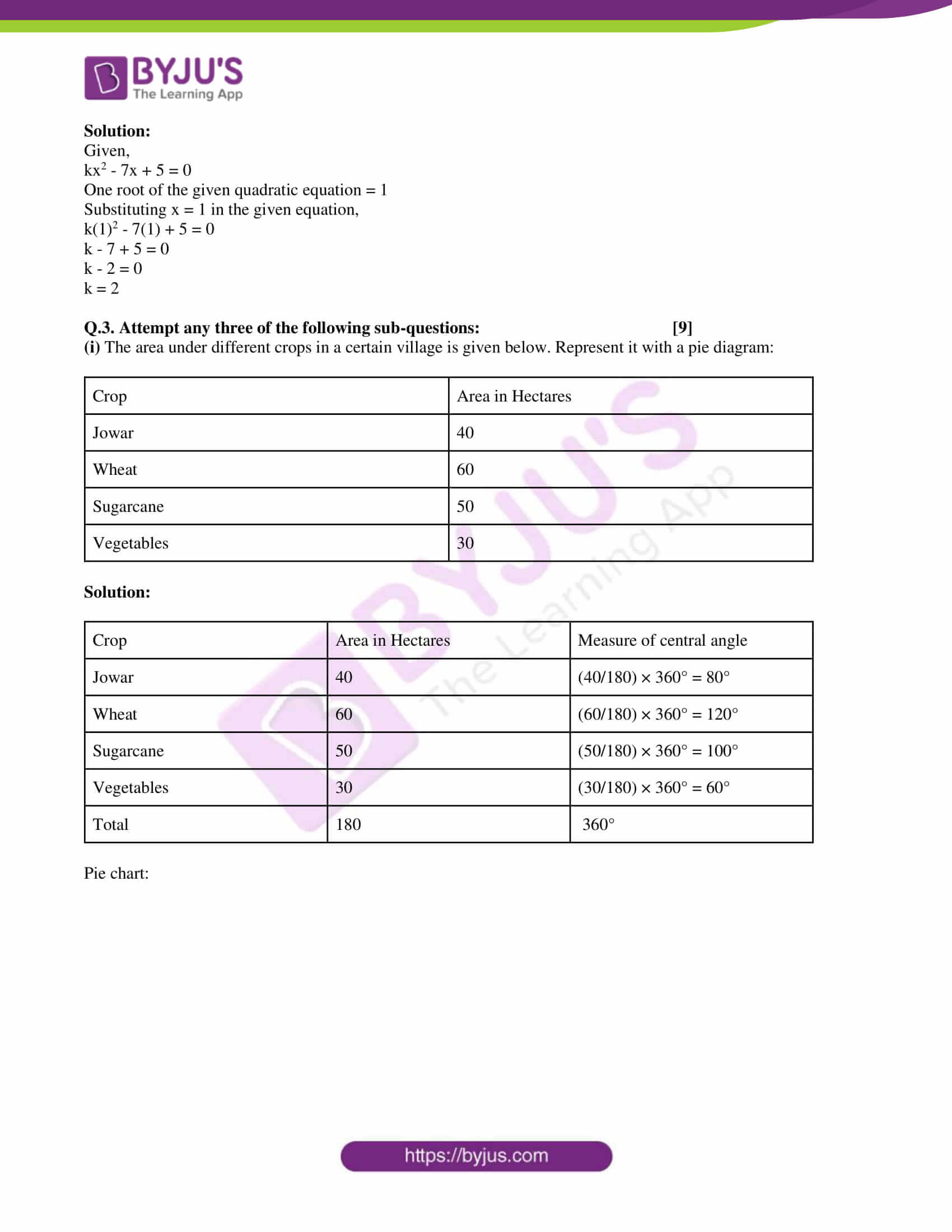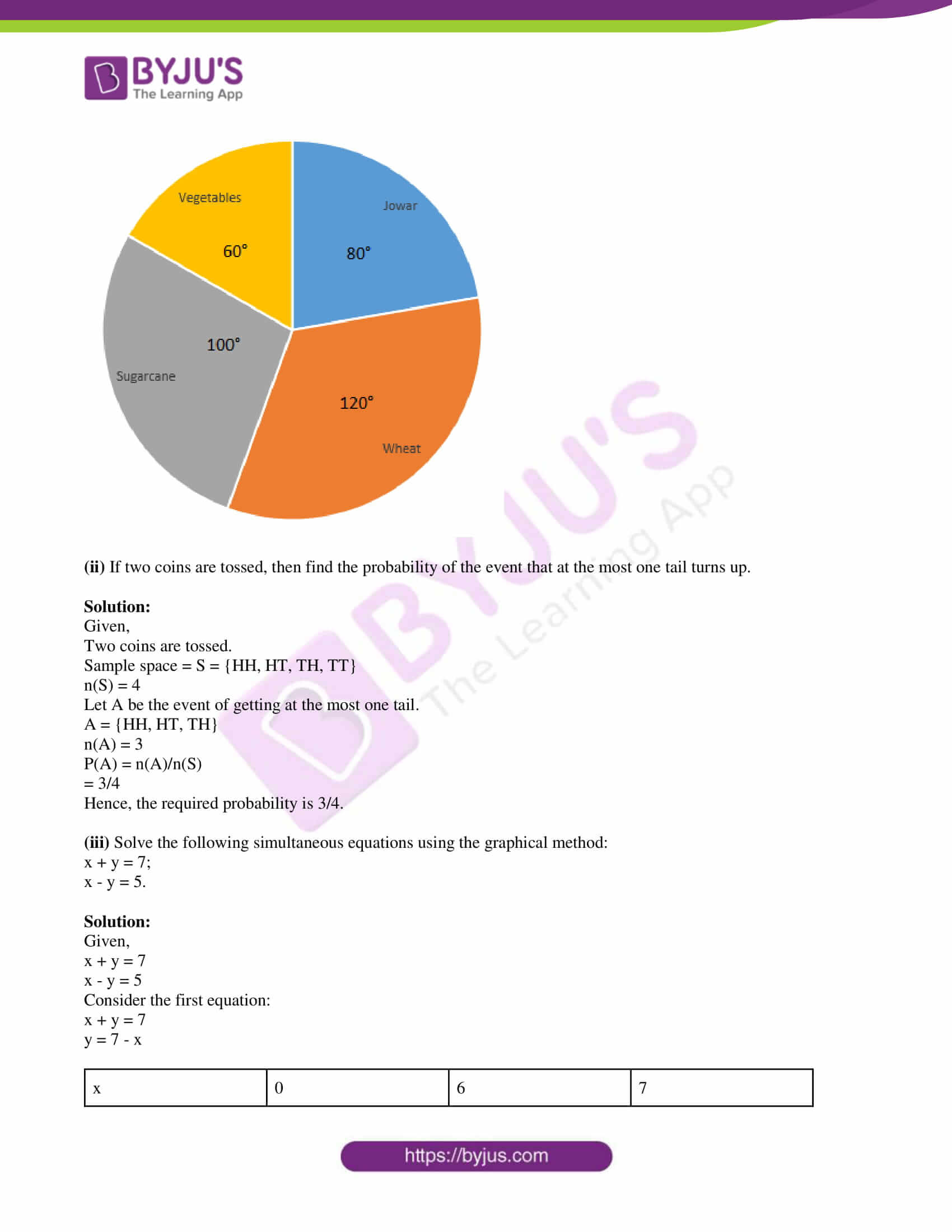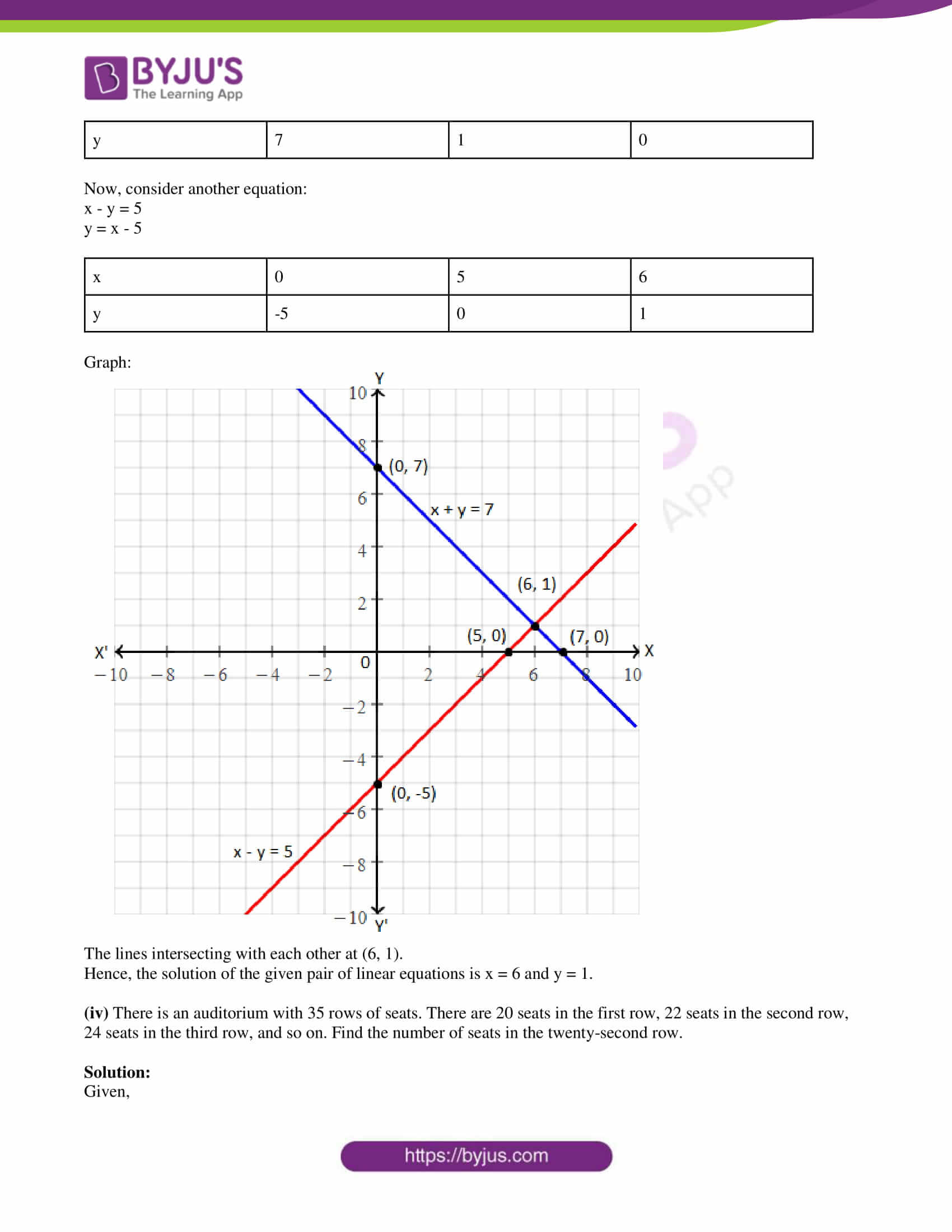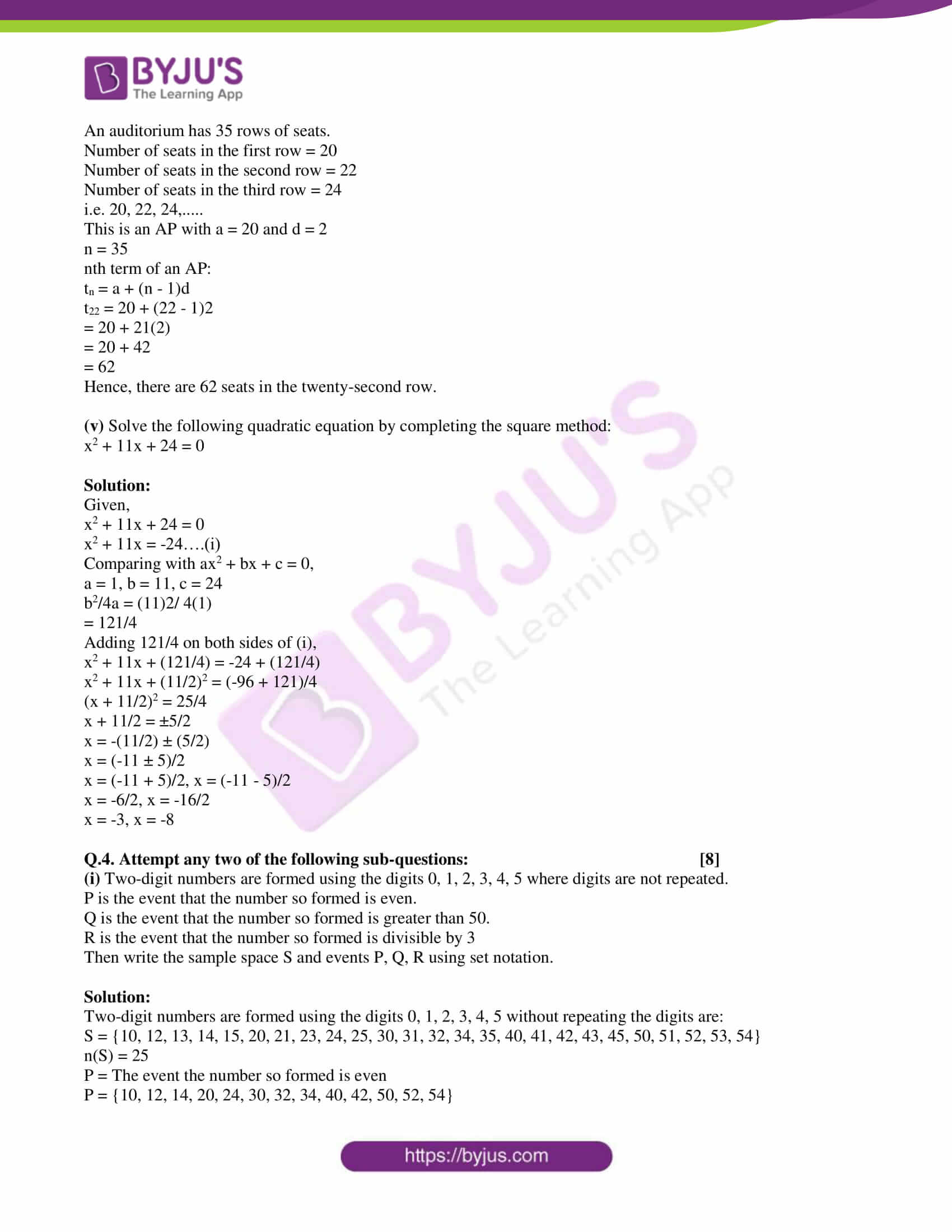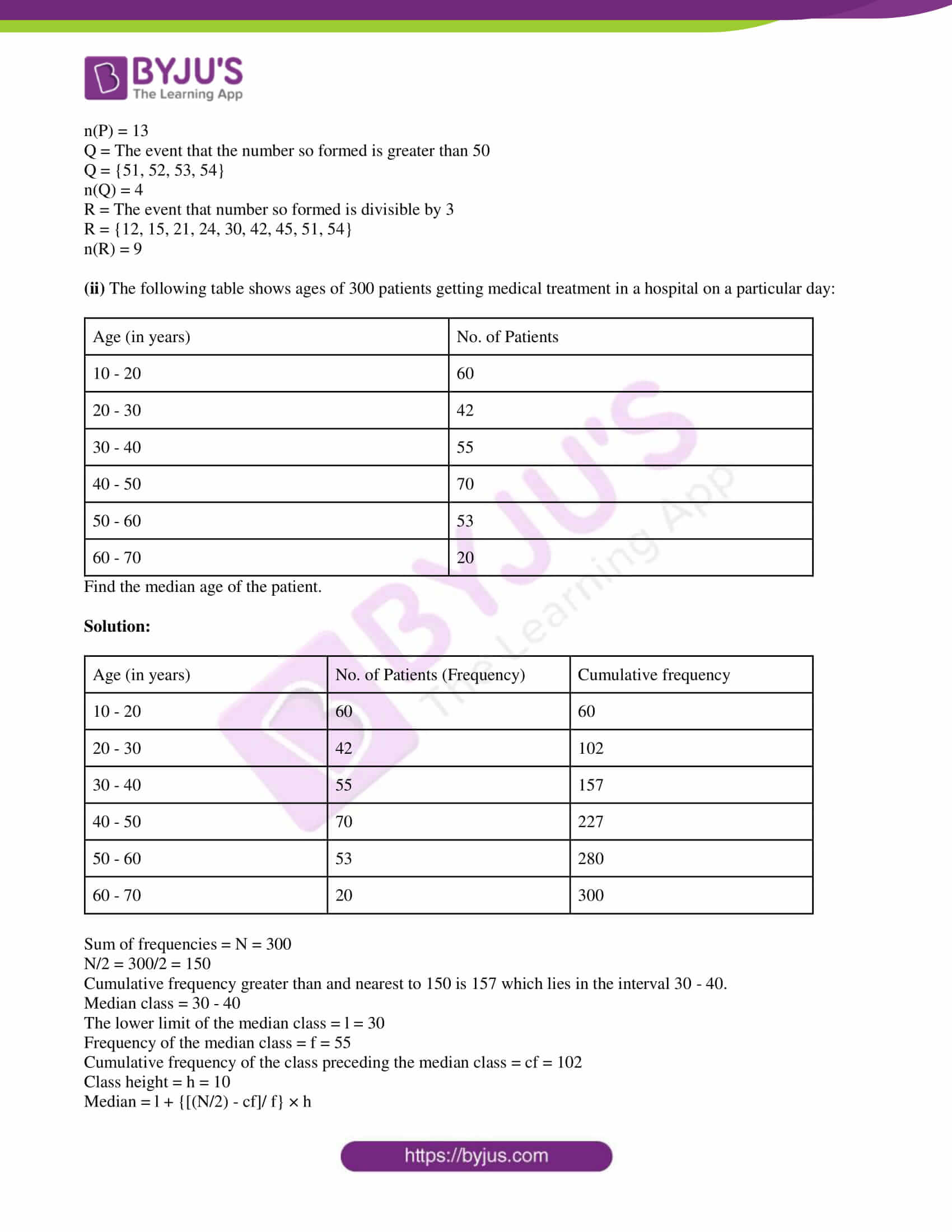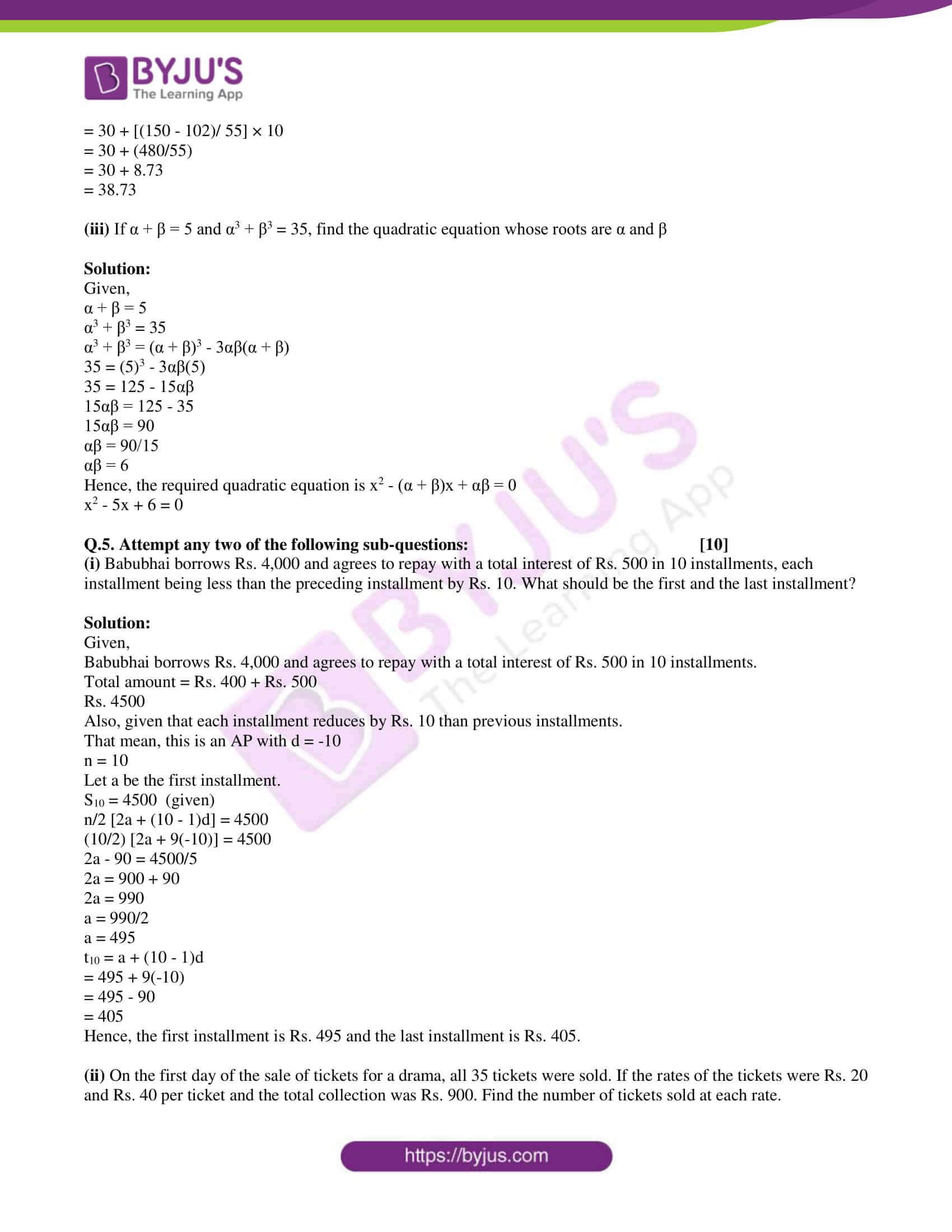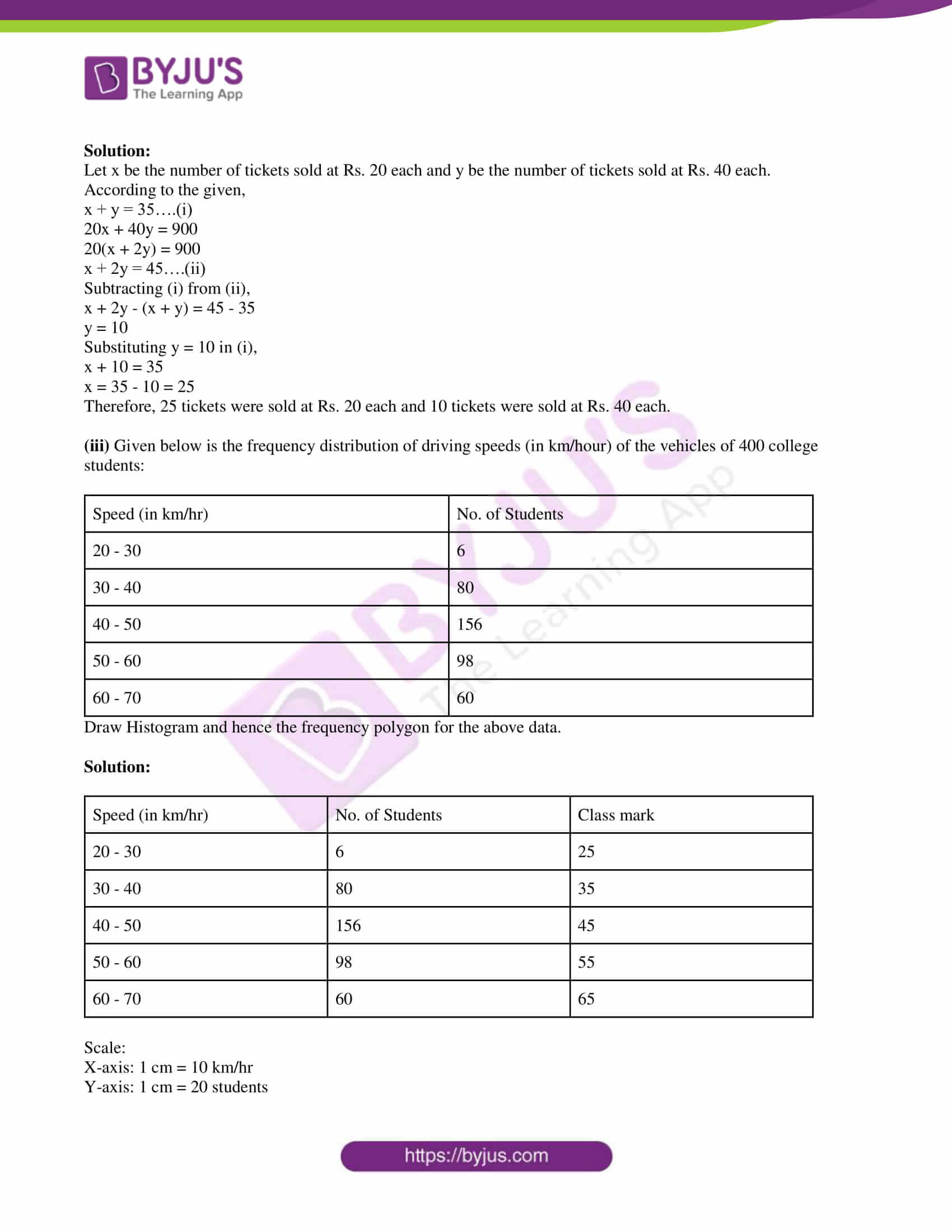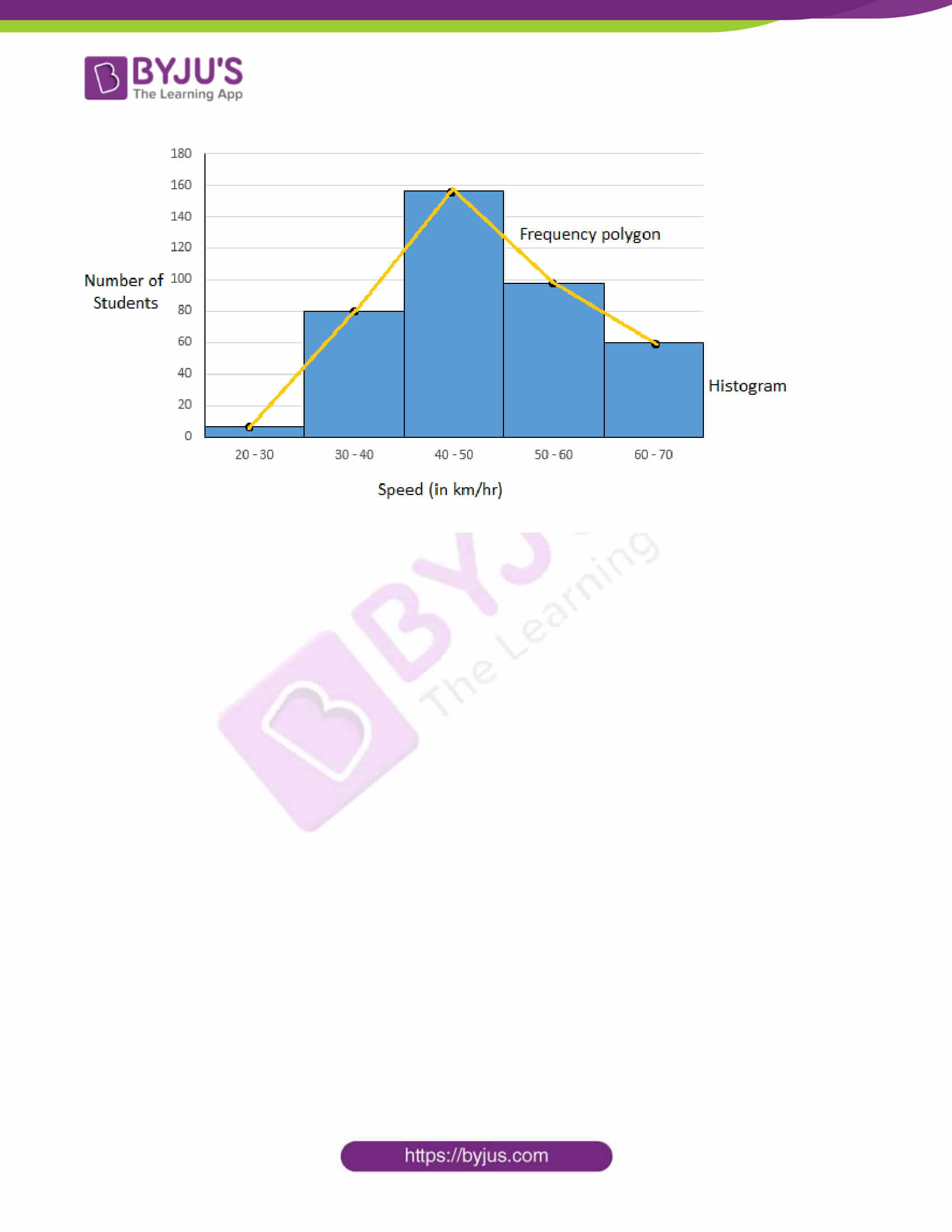PART – A

Q. 1. Attempt any five of the following sub-questions: 

(i) State whether the following sequence is an A.P. or not?

1, 4, 7, 10,….

Solution:

Given,

1, 4, 7, 10,….

First term = 1

Second term – First term = 4 – 1 = 3

Third term – Second term = 7 – 4 = 3

The common difference is the same throughout the sequence.

Hence, the given sequence is an Arithmetic progression.

(ii) A card is drawn from the pack of 25 cards labeled with numbers 1 to 25. Write the sample space for this random experiment.

Solution:

Given,

25 cards labeled with numbers from 1 to 25.

Sample space = S = {1, 2, 3, 4, 5, ….., 25}

n(S) = 25

(iii) Find the value of x + y, if

12x + 13y = 29 and

13x + 12y = 21

Solution:

Given,

12x + 13y = 29….(i)

13x + 12y = 21….(ii)

12x + 13y + 13x + 12y = 29 + 21

25x + 25y = 50

25(x + y) = 50

x + y = 50/25

x + y = 2

(iv) For a sequence, if Sn = n/(n + 1) then find the value of S10.

Solution:

Given,

Sn = n/(n + 1)

S10 = 10/ (10 + 1)

= 10/11

(v) Verify whether 1 is the root of the quadratic equation:

x2 + 3x – 4 = 0.

Solution:

If α is the root of the quadratic equation f(x) = 0, then f(α) = 0.

Given,

x2 + 3x – 4 = 0

Substituting x = 1,

LHS = (1)2 + 3(1) – 4

= 1 + 3 – 4

= 0

= RHS

Hence, 1 is the root of the given quadratic equation.

(vi) If x + y = 5 and x = 3, then find the value of y.

Solution:

Given,

x + y = 5….(i)

x = 3

Substituting x = 3 in (i),

3 + y = 5

y = 5 – 3

y = 2

Q.2. Attempt any four of the following sub-questions: 

(i) Solve the following quadratic equation by factorization method

x2 – 7x + 12 = 0.

Solution:

Given,

x2 – 7x + 12 = 0

Using the factorization method: splitting the middle term

x2 – 3x – 4x + 12 = 0

x(x – 3) – 4(x – 3) = 0

(x – 4)(x – 3) = 0

x – 4 = 0, x – 3 = 0

x = 4, x = 3

(ii) Find the term t10 of an A.P.:

4, 9, 14,….

Solution:

Given AP:

4, 9, 14,….

First term = a = 4

Common difference = d = 9 – 4 = 5

nth term of an AP,

tn = a + (n – 1)d

t10 = 4 + (10 – 1)5

= 4 + 9(5)

= 4 + 45

= 49

Therefore, t10 = 49.

(iii) If point A(2, 3) lies on the graph of the equation 5x + ay = 19, then find a.

Solution:

Given,

A(2, 3) lies on the graph of the equation 5x + ay = 19.

That means, the point satisfies the given equation.

Substituting x = 2 and y = 3 in the given linear equation,

5(2) + a(3) = 19

10 + 3a = 19

3a = 19 – 10

3a = 9

a = 9/3

a = 3

(iv) A die is thrown. If A is an event of getting an odd number, then write the sample space and event A in set notation.

Solution:

Given,

A die is thrown.

Sample space = S = {1, 2, 3, 4, 5, 6}

n(S) = 6

A = The event of getting an odd number

A = {1, 3, 5}

n(A) = 3

(v) For a certain frequency distribution, the value of Mean is 101 and Median is 100. Find the value of Mode.

Solution:

Given,

Mean = 101

Median = 100

We know that,

Mean – Mode = 3(Mean – Median)

101 – Mode = 3(101 – 100)

101 – Mode = 3(1)

101 – 3 = Mode

⇒ Mode = 98

(vi) If one root of the quadratic equation kx2 – 7x + 5 = 0 is 1, then find the value of k.

Solution:

Given,

kx2 – 7x + 5 = 0

One root of the given quadratic equation = 1

Substituting x = 1 in the given equation,

k(1)2 – 7(1) + 5 = 0

k – 7 + 5 = 0

k – 2 = 0

k = 2

Q.3. Attempt any three of the following sub-questions: 

(i) The area under different crops in a certain village is given below. Represent it with a pie diagram:

 Crop Area in Hectares Jowar 40 Wheat 60 Sugarcane 50 Vegetables 30

Solution:

 Crop Area in Hectares Measure of central angle Jowar 40 (40/180) × 360° = 80° Wheat 60 (60/180) × 360° = 120° Sugarcane 50 (50/180) × 360° = 100° Vegetables 30 (30/180) × 360° = 60° Total 180 360°

Pie chart: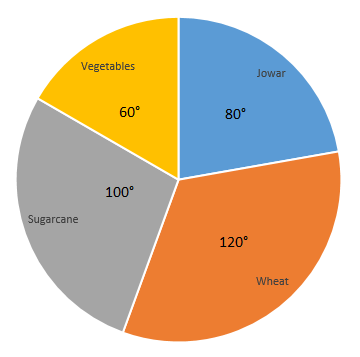(ii) If two coins are tossed, then find the probability of the event that at the most one tail turns up.

Solution:

Given,

Two coins are tossed.

Sample space = S = {HH, HT, TH, TT}

n(S) = 4

Let A be the event of getting at the most one tail.

A = {HH, HT, TH}

n(A) = 3

P(A) = n(A)/n(S)

= 3/4

Hence, the required probability is 3/4.

(iii) Solve the following simultaneous equations using the graphical method:

x + y = 7;

x – y = 5.

Solution:

Given,

x + y = 7

x – y = 5

Consider the first equation:

x + y = 7

y = 7 – x

 x 0 6 7 y 7 1 0

Now, consider another equation:

x – y = 5

y = x – 5

 x 0 5 6 y -5 0 1

Graph: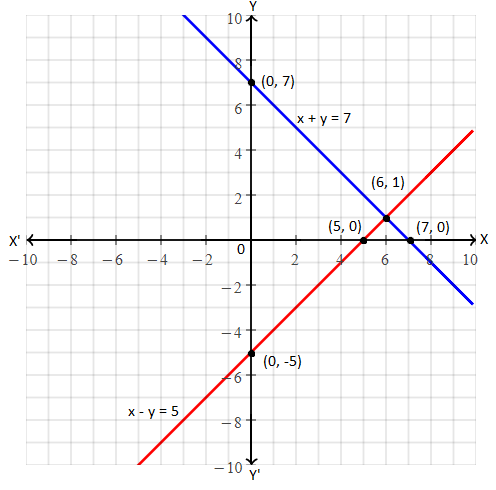The lines intersecting with each other at (6, 1).

Hence, the solution of the given pair of linear equations is x = 6 and y = 1.

(iv) There is an auditorium with 35 rows of seats. There are 20 seats in the first row, 22 seats in the second row, 24 seats in the third row, and so on. Find the number of seats in the twenty-second row.

Solution:

Given,

An auditorium has 35 rows of seats.

Number of seats in the first row = 20

Number of seats in the second row = 22

Number of seats in the third row = 24

i.e. 20, 22, 24,…..

This is an AP with a = 20 and d = 2

n = 35

nth term of an AP:

tn = a + (n – 1)d

t22 = 20 + (22 – 1)2

= 20 + 21(2)

= 20 + 42

= 62

Hence, there are 62 seats in the twenty-second row.

(v) Solve the following quadratic equation by completing the square method:

x2 + 11x + 24 = 0

Solution:

Given,

x2 + 11x + 24 = 0

x2 + 11x = -24….(i)

Comparing with ax2 + bx + c = 0,

a = 1, b = 11, c = 24

b2/4a = (11)2/ 4(1)

= 121/4

Adding 121/4 on both sides of (i),

x2 + 11x + (121/4) = -24 + (121/4)

x2 + 11x + (11/2)2 = (-96 + 121)/4

(x + 11/2)2 = 25/4

x + 11/2 = ±5/2

x = -(11/2) ± (5/2)

x = (-11 ± 5)/2

x = (-11 + 5)/2, x = (-11 – 5)/2

x = -6/2, x = -16/2

x = -3, x = -8

Q.4. Attempt any two of the following sub-questions: 

(i) Two-digit numbers are formed using the digits 0, 1, 2, 3, 4, 5 where digits are not repeated.

P is the event that the number so formed is even.

Q is the event that the number so formed is greater than 50.

R is the event that the number so formed is divisible by 3

Then write the sample space S and events P, Q, R using set notation.

Solution:

Two-digit numbers are formed using the digits 0, 1, 2, 3, 4, 5 without repeating the digits are:

S = {10, 12, 13, 14, 15, 20, 21, 23, 24, 25, 30, 31, 32, 34, 35, 40, 41, 42, 43, 45, 50, 51, 52, 53, 54}

n(S) = 25

P = The event the number so formed is even

P = {10, 12, 14, 20, 24, 30, 32, 34, 40, 42, 50, 52, 54}

n(P) = 13

Q = The event that the number so formed is greater than 50

Q = {51, 52, 53, 54}

n(Q) = 4

R = The event that number so formed is divisible by 3

R = {12, 15, 21, 24, 30, 42, 45, 51, 54}

n(R) = 9

(ii) The following table shows ages of 300 patients getting medical treatment in a hospital on a particular day:

 Age (in years) No. of Patients 10 – 20 60 20 – 30 42 30 – 40 55 40 – 50 70 50 – 60 53 60 – 70 20

Find the median age of the patient.

Solution:

 Age (in years) No. of Patients (Frequency) Cumulative frequency 10 – 20 60 60 20 – 30 42 102 30 – 40 55 157 40 – 50 70 227 50 – 60 53 280 60 – 70 20 300

Sum of frequencies = N = 300

N/2 = 300/2 = 150

Cumulative frequency greater than and nearest to 150 is 157 which lies in the interval 30 – 40.

Median class = 30 – 40

The lower limit of the median class = l = 30

Frequency of the median class = f = 55

Cumulative frequency of the class preceding the median class = cf = 102

Class height = h = 10

Median = l + {[(N/2) – cf]/ f} × h

= 30 + [(150 – 102)/ 55] × 10

= 30 + (480/55)

= 30 + 8.73

= 38.73

(iii) If α + β = 5 and α3 + β3 = 35, find the quadratic equation whose roots are α and β

Solution:

Given,

α + β = 5

α3 + β3 = 35

α3 + β3 = (α + β)3 – 3αβ(α + β)

35 = (5)3 – 3αβ(5)

35 = 125 – 15αβ

15αβ = 125 – 35

15αβ = 90

αβ = 90/15

αβ = 6

Hence, the required quadratic equation is x2 – (α + β)x + αβ = 0

x2 – 5x + 6 = 0

Q.5. Attempt any two of the following sub-questions: 

(i) Babubhai borrows Rs. 4,000 and agrees to repay with a total interest of Rs. 500 in 10 installments, each installment being less than the preceding installment by Rs. 10. What should be the first and the last installment?

Solution:

Given,

Babubhai borrows Rs. 4,000 and agrees to repay with a total interest of Rs. 500 in 10 installments.

Total amount = Rs. 400 + Rs. 500

Rs. 4500

Also, given that each installment reduces by Rs. 10 than previous installments.

That mean, this is an AP with d = -10

n = 10

Let a be the first installment.

S10 = 4500 (given)

n/2 [2a + (10 – 1)d] = 4500

(10/2) [2a + 9(-10)] = 4500

2a – 90 = 4500/5

2a = 900 + 90

2a = 990

a = 990/2

a = 495

t10 = a + (10 – 1)d

= 495 + 9(-10)

= 495 – 90

= 405

Hence, the first installment is Rs. 495 and the last installment is Rs. 405.

(ii) On the first day of the sale of tickets for a drama, all 35 tickets were sold. If the rates of the tickets were Rs. 20 and Rs. 40 per ticket and the total collection was Rs. 900. Find the number of tickets sold at each rate.

Solution:

Let x be the number of tickets sold at Rs. 20 each and y be the number of tickets sold at Rs. 40 each.

According to the given,

x + y = 35….(i)

20x + 40y = 900

20(x + 2y) = 900

x + 2y = 45….(ii)

Subtracting (i) from (ii),

x + 2y – (x + y) = 45 – 35

y = 10

Substituting y = 10 in (i),

x + 10 = 35

x = 35 – 10 = 25

Therefore, 25 tickets were sold at Rs. 20 each and 10 tickets were sold at Rs. 40 each.

(iii) Given below is the frequency distribution of driving speeds (in km/hour) of the vehicles of 400 college students:

 Speed (in km/hr) No. of Students 20 – 30 6 30 – 40 80 40 – 50 156 50 – 60 98 60 – 70 60

Draw Histogram and hence the frequency polygon for the above data.

Solution:

 Speed (in km/hr) No. of Students Class mark 20 – 30 6 25 30 – 40 80 35 40 – 50 156 45 50 – 60 98 55 60 – 70 60 65

Scale:

X-axis: 1 cm = 10 km/hr

Y-axis: 1 cm = 20 students